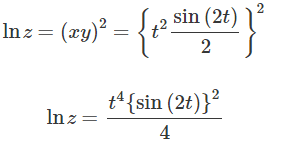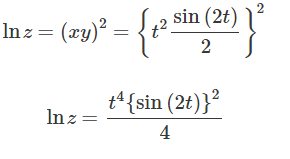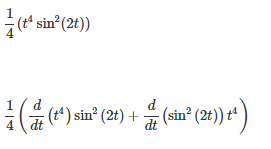# Differential Calculus Question

If z = e ^ (xy ^ 2), x = tcost, and y = tsint compute dx / dt for t = pi / 2

I kind of lost in this difficult question pls help

I tried putting down the xy but using ln

lnz = xy^2

Product rule? Or what. This is my first time encountering this kind of question

Last edited:

## Answers and Replies

Are you sure that you need to calculate ##\frac{dx}{dt}##? If so, then the first and 3rd equations are irrelevant - just calculate ##\frac{d}{dt} (t cost)## using the product rule, and plug in ##\frac π {2}## for ##t## into the derivative.

Are you sure that you need to calculate ##\frac{dx}{dt}##? If so, then the first and 3rd equations are irrelevant - just calculate ##\frac{d}{dt} (t cost)## using the product rule, and plug in ##\frac π {2}## for ##t## into the derivative.

Hmm that make sense
but in my hw it is dx/dt idk if that is a correction

I also think it is a dz/dt

Hmm that make sense
but in my hw it is dx/dt idk if that is a correction

I also think it is a dz/dt
For dz/dt, why don't you substitute the values of x and y in terms of t for the expression of z first?

For dz/dt, why don't you substitute the values of x and y in terms of t for the expression of z first?

hmmmm I tried thisProduct rule?

HallsofIvy
Science Advisor
Homework Helper
If the question is just "what is dx/dt", then the answer is just d(t cos(t))/dt= cos(t)- t sin(t), by the product rule.

But perhaps the problem was to find dz/dt and you are asked to find dx/dt as part of that.

By the "chain rule", $dz/dt= (\partial z/\partial x)(dx/dt)+ (\partial z/\partial y)(dy/dt)$

Since $z= e^{xy^2}$, $\partial z/\partial x= y^2e^{xy^2}$ and $\partial z/\partial y= 2xy e^{xy^2}$

As before, $dx/dt= cos(t)- t sin(t)$ and, since $y= t sin(t)$, $dy/dt= sin(t)+ t cos(t)$.

hmmmm I tried thisProduct rule?
There are two ways to proceed from here. Are you familiar with logarithmic differentiation? If yes, then just differentiate the left side with respect to t using chain rule, and the right side in the regular product rule manner.

If not, then convert this back into exponential form and use chain rule in combination with the product rule by letting the exponent equal to a new intermediate variable, such as u.

There are two ways to proceed from here. Are you familiar with logarithmic differentiation? If yes, then just differentiate the left side with respect to t using chain rule, and the right side in the regular product rule manner.

If not, then convert this back into exponential form and use chain rule in combination with the product rule by letting the exponent equal to a new intermediate variable, such as u.
I think I can do the Logarithmic Differentiation

d/dx(lnu) = 1/u * du/dx ??

I think I can do the Logarithmic Differentiation

d/dx(lnu) = 1/u * du/dx ??
This is true for the LHS, except that instead of u there will be a z, and instead of x we will have a t - there is no need to introduce a new variable for differentiating the LHS.

This is true for the LHS, except that instead of u there will be a z, and instead of x we will have a t - there is no need to introduce a new variable for differentiating the LHS.
Hmm can you lead me there? I think I can understand if I can grasp more of it thank you!

Hmm can you lead me there? I think I can understand if I can grasp more of it thank you!
You already did that. The equation becomes $$\frac 1 {z} \frac{dz}{dt} = \frac{d}{dt} (\frac{t^4 (sin 2t)^2}{4})$$ , and you can proceed from here by applying the product rule.

Last edited:
You already did that. The equation becomes $$\frac 1 {z} \frac{dz}{dt} = \frac{d}{dt} (\frac{t^4 (sin 2t)^2}{4})$$ , and you can proceed from here by applying the chain rule.

I'll chain rule the RHS?
I'll took the constant out first so
1/4(t^4(sin2t)^2)?
I don't know if that's what you meant

I'll chain rule the RHS?
I'll took the constant out first so
1/4(t^4(sin2t)^2)?
I don't know if that's what you meant
Oh, my bad. I meant use the product rule.

Oh, my bad. I meant use the product rule.

SooooooI'll just product rule this then substitue pi/2?

Yes.

Yes.

I got 0.3448 is it right?

I got 0.3448 is it right?

Are you sure that works out to give 0.3448 ?

So did you get an answer?

So did you get an answer?

I got 0.3448 I don't know if the answer is correct but at least I understand the concept which is more important so thank you mates!

I got 0.3448 I don't know if the answer is correct but at least I understand the concept which is more important so thank you mates!
EDIT: The answer is close, but not 0.3448 . Can show the derivative that you got?

Last edited:
I got 0.3448 I don't know if the answer is correct but at least I understand the concept which is more important so thank you mates!

I think your answer is wrong . How then , can you say this ?